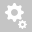##Recently viewed tickets• Sales
• Library Model Request
• Technical Support

# What is the difference between a Comprehensive Study and a ANSI study?

ANSI fault follows ANSI C37 standards for calculating equipment ratings. The comprehensive fault follows a traditional Ohm’s Law calculation for fault current.

For Low voltage systems, the calculations are the same except for two items:
1) ANSI calculates the X/R ratios based on separate X and R networks whereas Comprehensive uses a single complex network.
2) ANSI uses the X/R/ ratio to adjust the calculated short circuit value to compare directly with molded case and power circuit breaker ratings based on ANSI test power factor. In other words, the calculated short circuit value is increased if the X/R/ ratio is larger that the ANSI test power factor.

For Medium and High Voltage systems: The momentary calculations are similar. The largest difference is in Interrupting Calculations: ANSI uses the ANSI C37 tables and curves to simulate AC and DC decay for interrupting calculations, whereas Comprehensive accounts for the system DC decay but ignores the AC machine decay in all cases.

http://www.skm.com/images/ANSI_DAPPER_Fault_Comparison.pdf